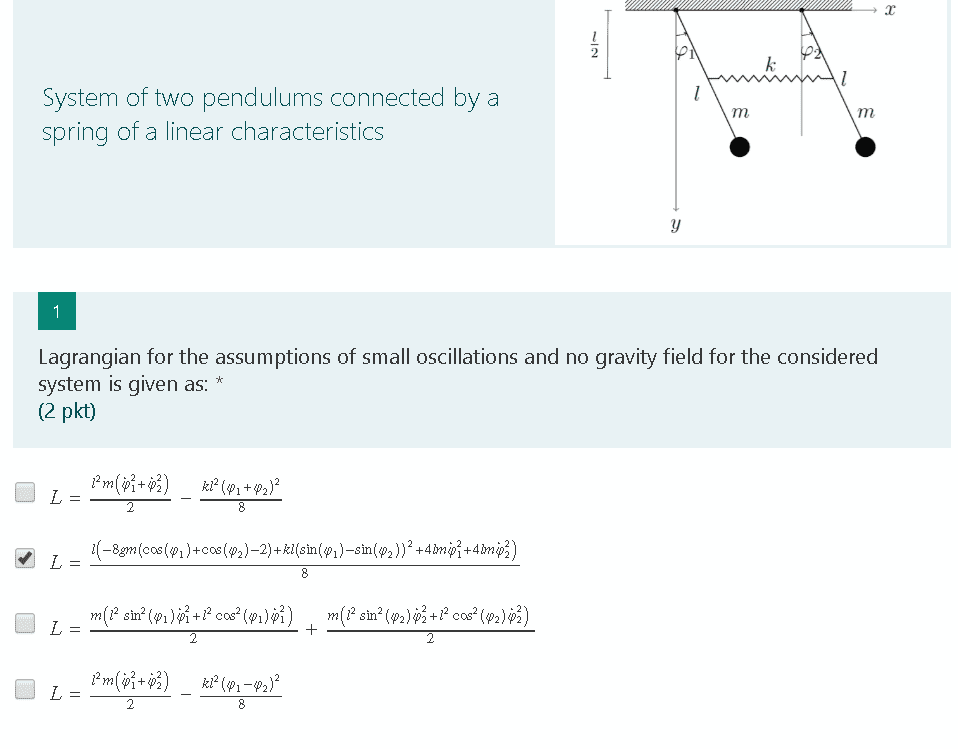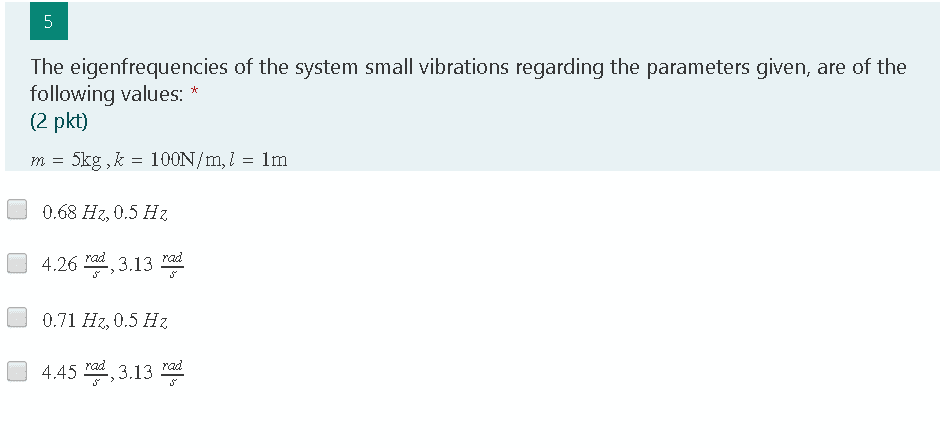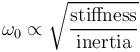# Engineering Homework problem - Pendulum oscillatory system

Homework Statement:
Mechanical Vibrations, #Lagrange #eigenfrequency
Relevant Equations:
Lagrange equations, eigenfrequency equation for the given systemHi All,

Anyone willing to help out in explaining what eigenfreuqncy for this oscilatory system, would be? Also if anybody knows the equation to calulate this stuff please, if you're willing to share I'd be greatful!

Thanks, regards.

Truthfully I'm not in possession of any course materials from vibration mechanisms. What I do have are educative videos, lectures provided by my lecturer and notes which I keep track of. I know that sucks but the rest I have to find out on my own which makes me anxious as I'm not sure sometimes if I'm even looking correctly.

So far I know how to calculate a 1DoF natural frequency with given parameters. For the MDoF system I have frankly no clue what should I even look for. Unfortunately, my professor hands out these Microsoft form tests before each lecture, but I'm not certain if I will learn anything during the lecture too, therefore I came here for help.

Cheers.

Also, the only potentially valid equation which I managed to find is as follows:BvU
Homework Helper
hands out these Microsoft form tests
Funny sheets, with a question 1 and then a question 5.
Funny they make a "no gravity field" limitation too.

Did you notice I asked a few questions ? They were aimed at you !

What is the small angle approximation for a pendulum ?

1. Small oscilations = vibrations were values of cos/sin(angles) may be approximated to angles [rad]. For cos(phi)=1, sin(phi)/tan(phi)=phi

BvU
Homework Helper
So what does that leave for question 1 ?

the first box should be checked instead of the 2nd one

BvU
Homework Helper
I agree that box 2 can be discarded (##g = 0##) and for box 2 and 3 the small angle approximations ##\sin x = x, \ \cos x = 1## have not been implemented.

Explain why you think that box one is better than the alternative ...

The difference between boxes 4 and 1 is the change in angular displacement for PE, where it varies with (+) and (-) sign for each answer. I believe that the correct solution should be one containing the element with (phi1-phi2) as we are superimposing one displacement onto the second one. That means that by exciting the first pendulum the impulse through the spring continues to flow until it reaches the second pendulum. The motion then becomes for the given moment unstable as the second pendulum is excited and the spring receives an impulse that is then directed in the negative direction (-phi2).

Last edited:
the first box should be checked instead of the 2nd one

so I meant box 4 not box 1

BvU